# Wave Nature of Electron

As a young student at the University of Paris, Louis DeBroglie had been impacted by relativity and the photoelectric effect, both of which had been introduced in his lifetime. The photoelectric effect pointed to the particle properties of light, which had been considered to be a wave phenomenon. He wondered if electrons and other "particles" might exhibit wave properties. The application of these two new ideas to light pointed to an interesting possibility: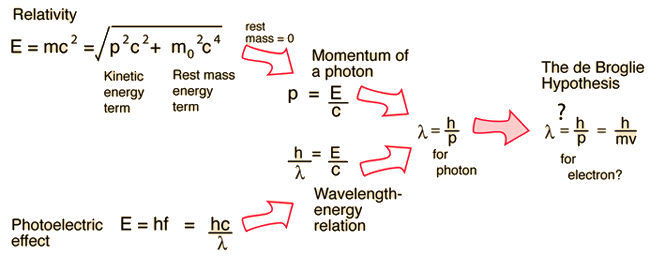### Confirmation of the DeBroglie hypothesis came in the Davisson- Germer experiment.

 Examples of electron waves
 deBroglie wavelength
 If the electron is a wave, what is waving?
Index

 HyperPhysics***** Quantum Physics R Nave
Go Back

# Examples of Electron Waves

Two specific examples supporting the wave nature of electrons as suggested in the DeBroglie hypothesis are the discrete atomic energy levels and the diffraction of electrons from crystal planes in solid materials. In the Bohr model of atomic energy levels, the electron waves can be visualized as "wrapping around" the circumference of an electron orbit in such a way as to experience constructive interference.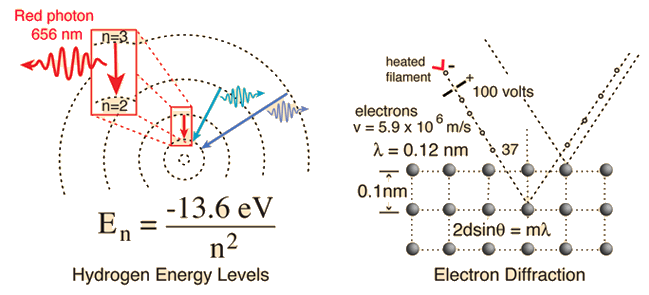Click on either example for further details.

The wave nature of the electron must be invoked to explain the behavior of electrons when they are confined to dimensions on the order of the size of an atom. This wave nature is used for the quantum mechanical "particle in a box" and the result of this calculation is used to describe the density of energy states for electrons in solids.

 If the electron is a wave, what is waving?
Index

 HyperPhysics***** Quantum Physics R Nave
Go Back

# DeBroglie Hypothesis

Suggested by De Broglie in about 1923, the path to the wavelength expression for a particle is by analogy to the momentum of a photon. Starting with the Einstein formula: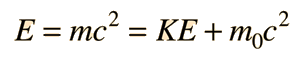Another way of expressing this is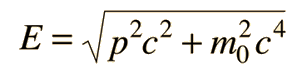Therefore, for a particle of zero rest mass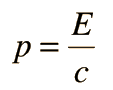For a photon:The momentum-wavelength relationship for a photon can then be derived and this DeBroglie wavelength relationship applies to other particles as well.### Calculation of DeBroglie wavelength

Index

 HyperPhysics***** Quantum Physics R Nave
Go Back

# DeBroglie Wavelengths

## The Davisson-Germer experiment showed that electrons exhibit the DeBroglie wavelength given by:If you explore the wavelength values for ordinary macroscopic objects like baseballs, you will find that their DeBroglie wavelengths are ridiculously small. Comparison of the power of ten for the wavelength will show that the wavelengths of ordinary objects are much smaller than a nucleus. The implication is that for ordinary objects, you will never see any evidence of their wave nature, and they can be considered to be particles for all practical purposes.

### Calculation

Index

 HyperPhysics***** Quantum Physics R Nave
Go Back

# DeBroglie Wavelengths

## The Davisson-Germer experiment showed that electrons exhibit the DeBroglie wavelength given by:The wavelength associated with a particle can be calculated from:

### wavelength = h/(mass x velocity)

 Click on the desired quantity after data entry.
 For a particle of mass x10^ kg
 ==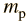traveling at velocity x10^ m/s
the associated DeBroglie wavelength is x10^m = m =nm.

You may enter values in any of the boxes. When you have finished entering data, click on the quantity you wish to calculate in the formula above. Wavelength values will be reconciled, but no further calculation will be done until you select which item you wish to calculate. Caution! This calculation is non-relativistic and accurate only for speeds much less than the speed of light. For speeds over a tenth of the speed of light a relativistic calculation should be used.

 If you explore the wavelength values for ordinary macroscopic objects like baseballs, you will find that their DeBroglie wavelengths are ridiculously small. Comparison of the power of ten for the wavelength will show what the wavelengths of ordinary objects are much smaller than a nucleus. The implication is that for ordinary objects, you will never see any evidence of their wave nature, and they can be considered to be particles for all practical purposes.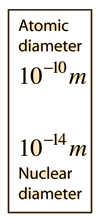### Alternate calculation of DeBroglie wavelength in terms of particle energy

Index

 HyperPhysics***** Quantum Physics R Nave
Go Back